# python一些小习题 田字格、isodd、isnum、multi、isprime、绝对素数、哥德巴赫猜想

2020-06-29 05:04 176 查看

## 1.1.定义函数输出一个简单的田字格。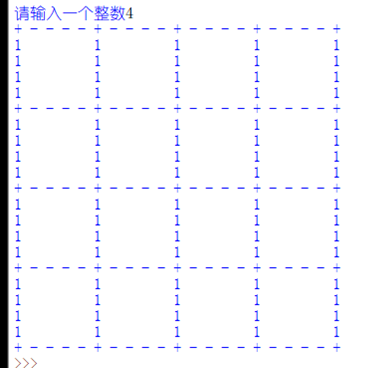```#田字格
a=int(input('请输入一个整数'))
b='- '*a+'+ '#空格真的坑死人
print('+ '+b*a)#第一行
for j in range(a):#后面的行
for i in range(a):
c='  '*a+'1 '
print('1 '+c*a)
d='- '*a+'+ '
print('+ '+d*a)```

```def bc(x):
print("+ ", end="")
for i in range(x):
print("- "*x+"+ " , end="")
print()
def zj(x):
for i in range(x):
print("1 " , end="")
for j in range(x):
print("  "*x+"1 ", end="")
print()
def main():
x=eval(input("请输入一个整数"))
bc(x)
for j in range(x):
zj(x)
bc(x)
main()```

## 2.实现isodd函数，

Lily的答案，等待命运的批判吧

```#isodd
def isodd(a):
io=bool(0)#这是我的个人风格啦，不喜欢直接True、False
if a%2!=0:
io=bool(1)
print('{}是奇数' .format(a))
else:
print('{}是偶数' .format(a))
return io
try:
a=eval(input("请输入一个整数"))
if type(a)==int:
#这估计和老师的写法就会不一样，我超喜欢type()==这种直接的函数
isodd(a)
except:
print("输入错误")```

```def isodd(x):
if x%2==1:
return True
else:
return False
try:
y=int(input("请输入一个整数"))
if isodd(y):
print("{}是奇数".format(y))
else:
print("{}是偶数".format(y))
except:
print("输入错误")```

## 3. 实现 isnum 函数，

（提示：通过输入异常实现）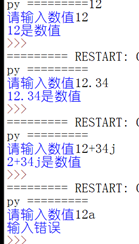```def isnum(x):
try:
if int(x):
#尽管不愿意承认，这的确简洁不少，好了，打算弃暗投明
return True
except:
try:
if float(x):
return True
except:
try: #try except嵌套
if complex(x):
return True
except:
return False
y=input("请输入数值")
if isnum(y): #把输出结果都放在主程序，会是个好习惯吧
print("{}是数值".format(y))
else:
print("输入错误")```

## 4. 实现multi函数，

```def multi(l):
mu=1
for i in l:
mu*=i
print (mu)

l=eval(input('请输入参数,用逗号分隔'))

multi(l)```

```def multi(*b):
s=1
for x in b:
s=s*x
return s
print(multi(2,3,4,5,6,7))```

## 5.实现isprime函数，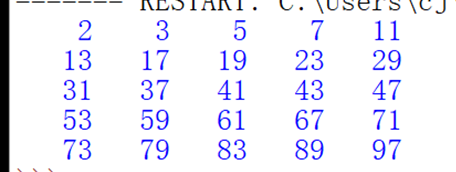```#isprime
def isprime(a):  #判断是不是素数
for i in range(2,a):  #从2开始
if a%i==0:
return False
else:
return True
c=0  #不要放在for循环里面，否则c每次都会被刷回0，起不到计数器的作作用
for j in range(2,100):
#因为j已经定在这个范围，所以j=1的情况不需要在上面的函数体现出来
if isprime(j):
print(str(j).rjust(5),end='')  #右对齐，不换行
c+=1
if c%5==0:  #够了5个，就换行
print(end = "\n")```

```def isprime(a):
try:   #又一次把异常处理放在函数里
for x in range(2,a):   #难得的与老师心连心
if a%x==0:
return False
return True
except:
print("输入格式错误")
k=0
for i in range(2,100):
if isprime(i):
k=k+1
print("{:5}".format(i),end="")
if k%5==0:
print()   #哦我忘了，print()自带换行效果```

## 6.绝对素数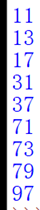```#绝对素数
def isprime(a):  #先判断是不是素数
for i in range(2,a):
if a%i==0:
return False
else:
return True

for j in range(10,100):  #所有两位数
J2=j//10 #分离出十位数
J1=j%10 #分离出个位数

j2=J2+J1*10 #求出颠倒之后的数
if isprime(j) and isprime(j2): #够素数，则绝对
print(j)```

```def isprime(a):
try:
for x in range(2,a):
if a%x==0:
return False
return True
except:
print("输入格式错误")
for i in range(2,100):
if isprime(i) and isprime(i%10*10+i//10):
#老师就是不一样，我哭了我这么多行
print(i)```

## 7.哥德巴赫猜想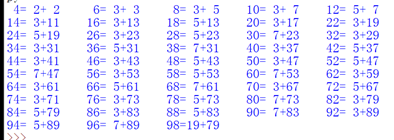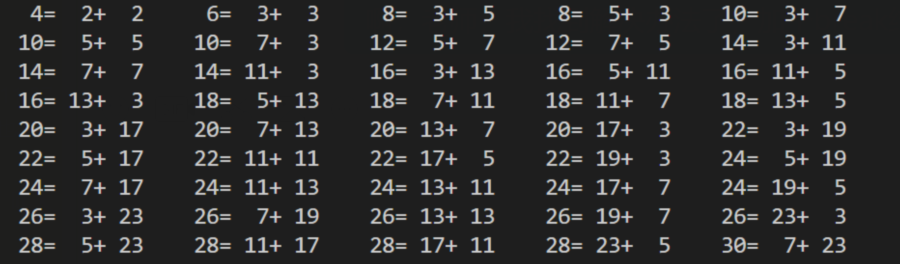``````clike
#哥德巴赫猜想
def isprime(a):
try:
for x in range(2,a):
if a%x==0:
return False
return True
except:
print("输入格式错误")
c=0
for i in range(4,100,2):
for j in range(2,i//2+1):
if isprime(j) and isprime(i-j):
print("{:2}={:2}+{:2}".format(i,j,i-j),end="    ")
c=c+1
if c%5==0:
print()
break```